# IB DP Physics: HL复习笔记6.2.3 Gravitational Field Strength

### Gravitational Field Strength

• There is a universal force of attraction between all matter with mass
• This force is known as the ‘force due to gravity’ or the weight
• The Earth’s gravitational field is responsible for the weight of all objects on Earth
• A gravitational field is defined as:

A region of space where a test mass experiences a force due to the gravitational attraction of another mass

•  The direction of the gravitational field is always towards the centre of the mass causing the field
• Gravitational forces cannot be repulsive
• Gravity has an infinite range, meaning it affects all objects in the universe
• There is a greater gravitational force around objects with a large mass (such as planets)
• There is a smaller gravitational force around objects with a small mass (almost negligible for atoms)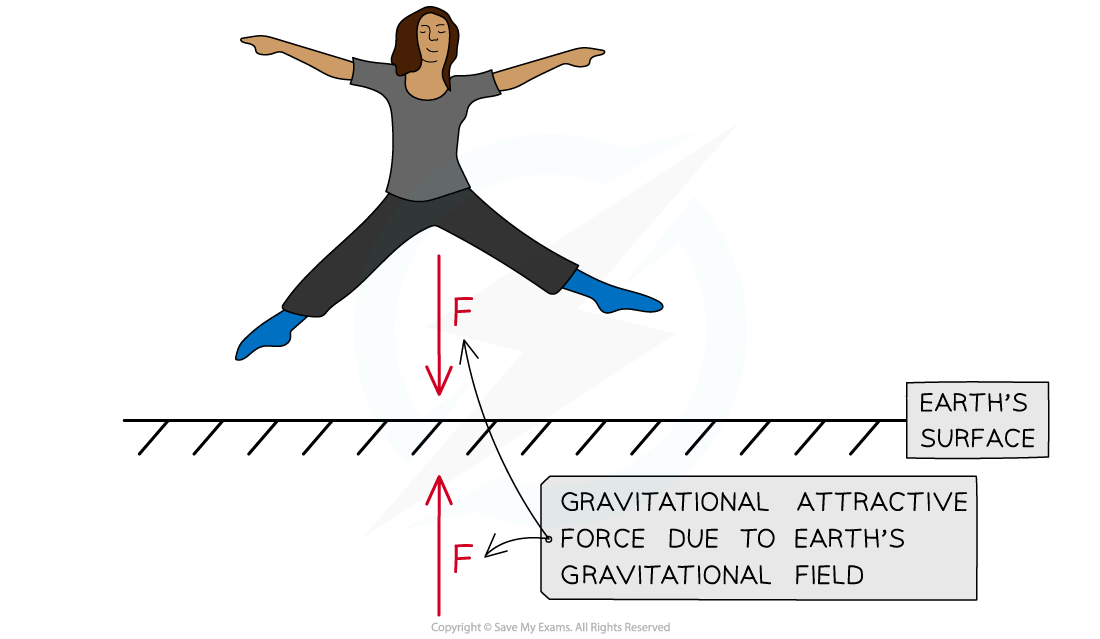The Earth's gravitational field produces an attractive force. The force of gravity is always attractive

• The gravitational field strength at a point is defined as:

The force per unit mass experienced by a test mass at that point

• This can be written in equation form as:• Where:
• g = gravitational field strength (N kg−1)
• F = force due to gravity, or weight (N)
• m = mass of test mass in the field (kg)
• This equation shows that:
• On planets with a large value of g, the gravitational force per unit mass is greater than on planets with a smaller value of g
• An object's mass remains the same at all points in space
• However, on planets such as Jupiter, the weight of an object will be greater than on a less massive planet, such as Earth
• This means the gravitational force would be so high that humans, for example, would not be unable to fully stand up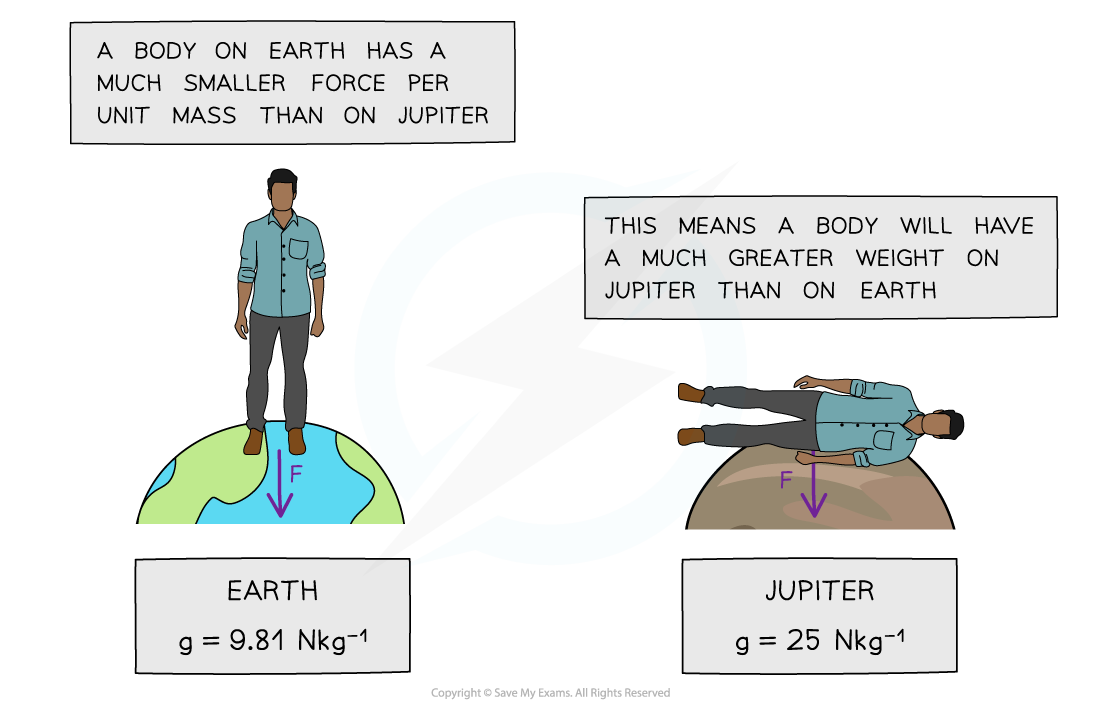A person’s weight on Jupiter would be so large that a human would be unable to fully stand up

• Factors that affect the gravitational field strength at the surface of a planet are:
• The radius (or diameter) of the planet
• The mass (or density) of the planet
• This can be shown by equating the equation F = mg with Newton's law of gravitation:• Substituting the force F with the gravitational force mg leads to:• Cancelling the mass of the test mass, m, leads to the equation:• Where:
• G = Newton's Gravitational Constant
• M = mass of the body causing the field (kg)
• r = distance from the mass where you are calculating the field strength (m)
• This equation shows that:
• The gravitational field strength g depends only on the mass of the body M causing the field
• Hence, objects with any mass m in that field will experience the same gravitational field strength
• The gravitational field strength g is inversely proportional to the square of the radial distance, r2

#### Worked Example

Calculate the mass of an object with weight 10 N on Earth.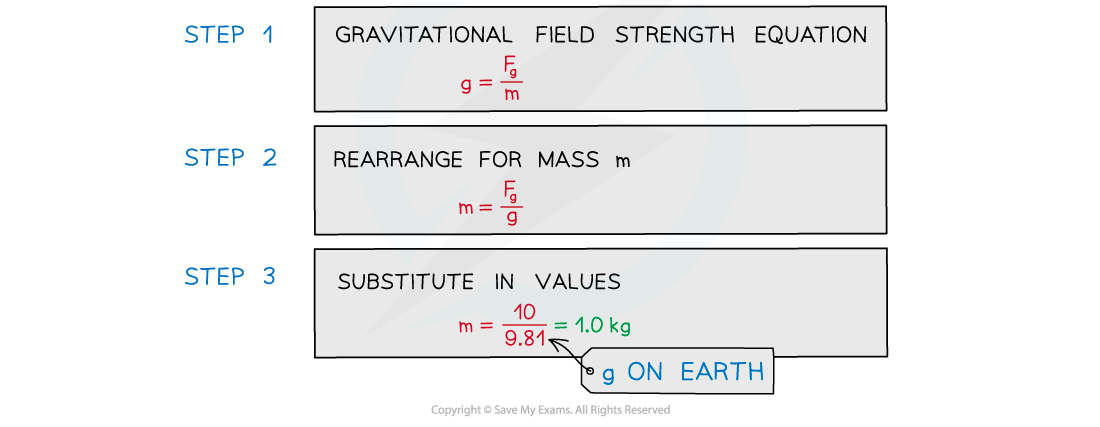#### Worked Example

The mean density of the Moon is 3/5 times the mean density of the Earth. The gravitational field strength on the Moon is 1/6 of the value on Earth.

Determine the ratio of the Moon's radius rM and the Earth's radius rE.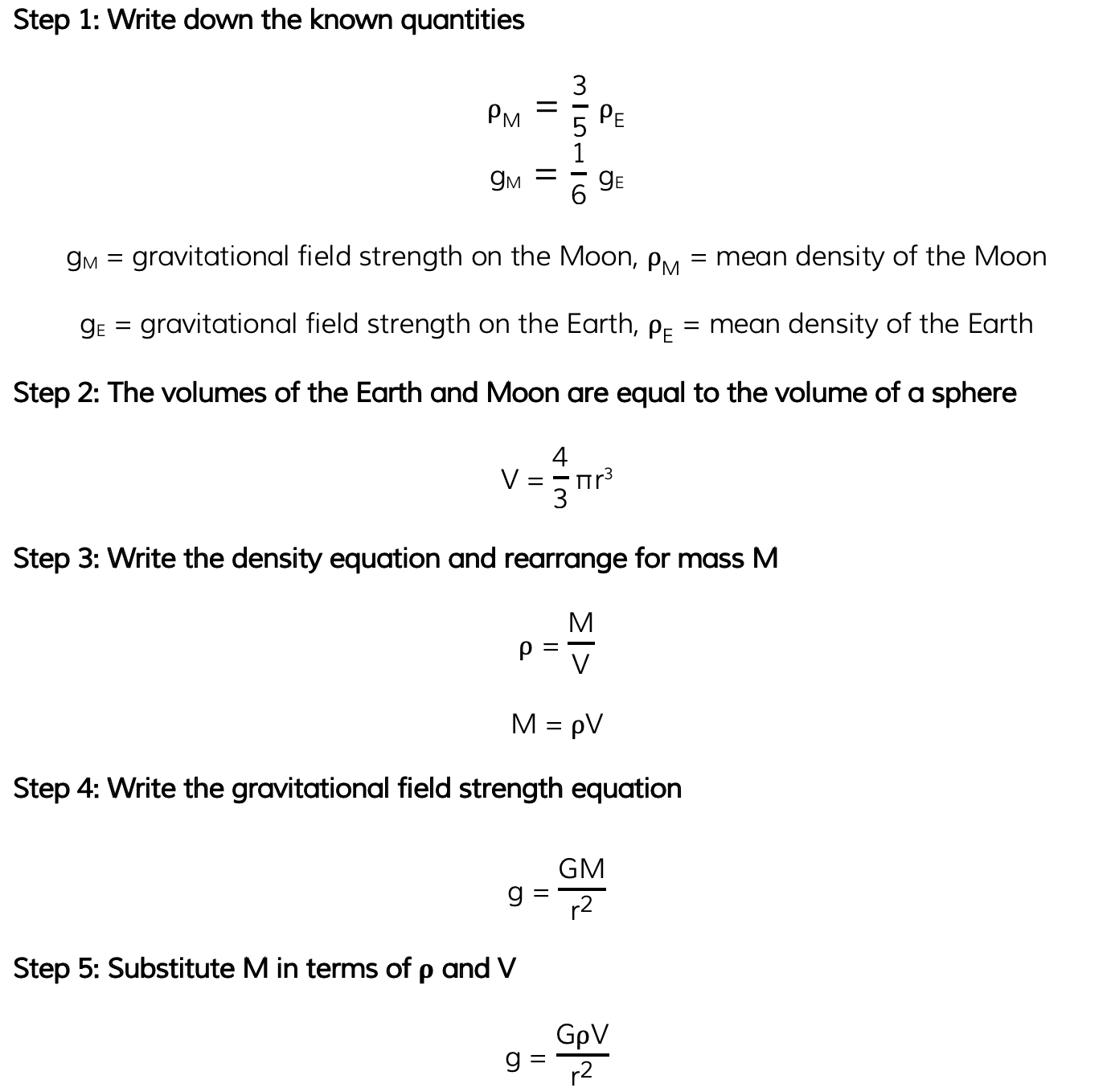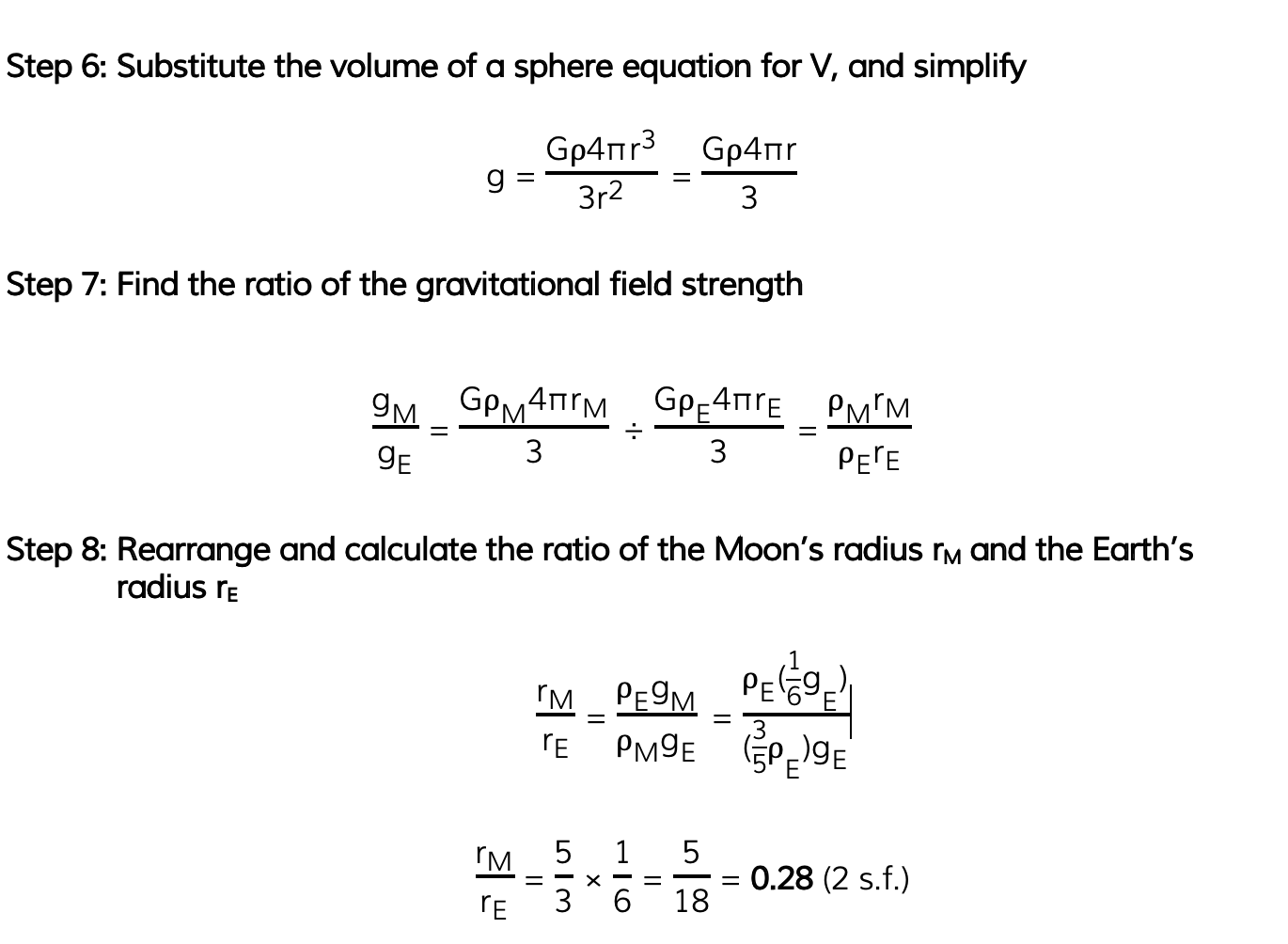#### Exam Tip

There is a big difference between g and G (sometimes referred to as ‘little g’ and ‘big G’ respectively), g is the gravitational field strength and G is Newton’s gravitational constant. Make sure not to use these interchangeably! Remember the equation density ρ = mass m ÷ volume V, which may come in handy with some calculations

### Resultant Gravitational Field Strength

• In a similar way to other vectors, such as force or velocity, the gravitational field strength due to two bodies can be determined
• This is because gravitational field strength is a vector, meaning it has both a magnitude and direction
• The resultant gravitational field strength is, therefore, the vector sum of the gravitational field strength due to each body

#### Worked Example

A planet is equidistant from two stars in a binary system. Each star has a mass of 5.0 × 1030 kg and the planet is at a distance of 3.0 × 1012 m from each star. Calculate the magnitude of the resultant gravitational field strength at the position of the planet.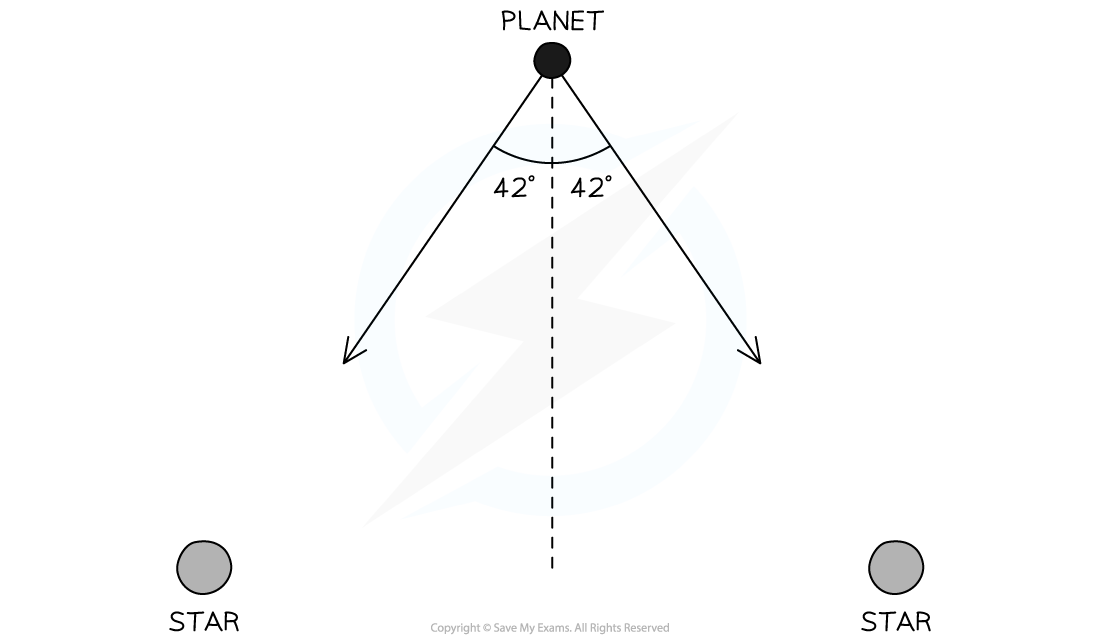Step 1: List the known quantities

• Mass of one star, M = 5.0 × 1030 kg
• Distance between one star and the planet, r = 3.0 × 1012 m
• Angle between the gravitational field strength and the planet, θ = 42°

Step 2: Write out the equation for gravitational field strengthStep 3: Calculate the gravitational field strength due to one starg = 3.7 × 10−5 N kg−1

Step 4: Resolve the vectors vertically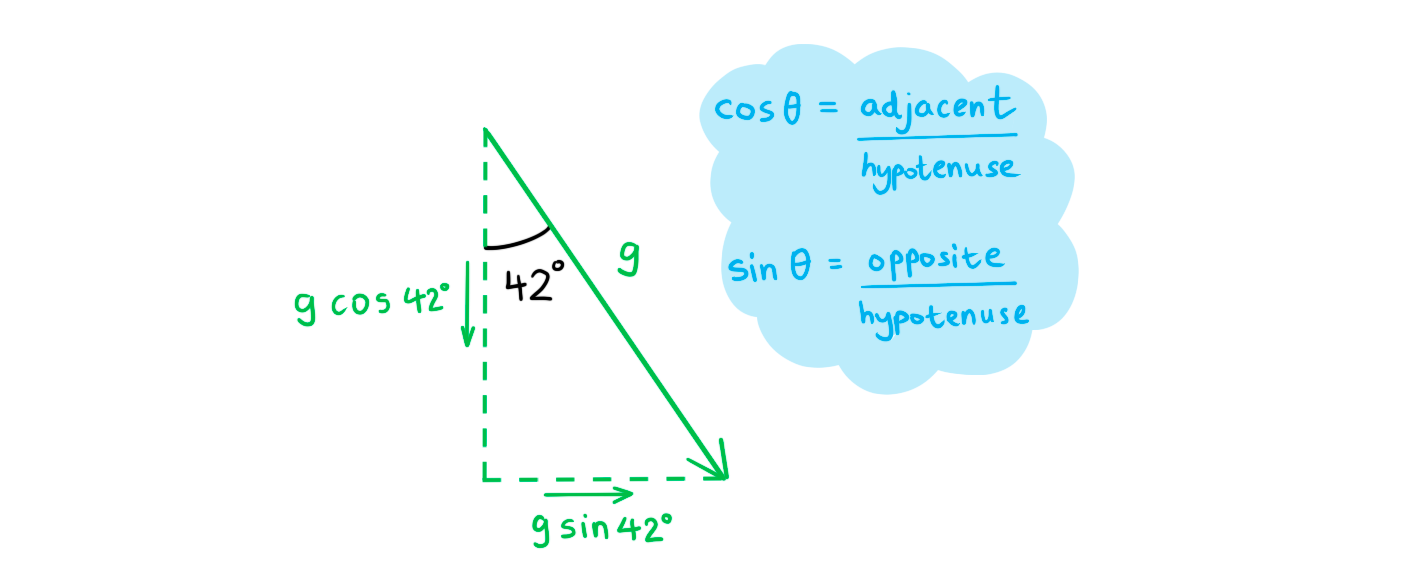• The vertical component of each vector is g cos 42°

Step 5: Use vector addition to determine the resultant gravitational field strength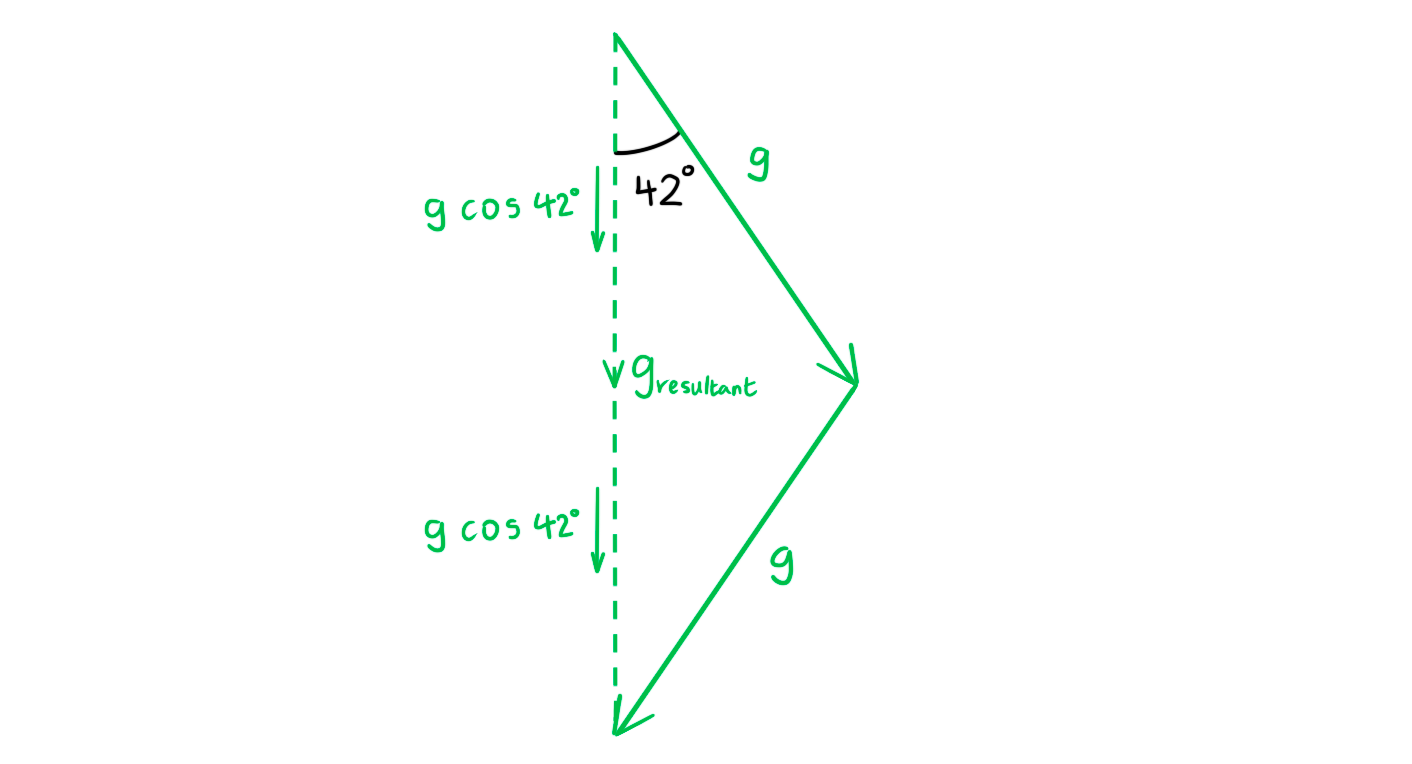gresultant = g cos 42° + g cos 42° = 2g cos 42°

gresultant = 2 × (3.7 × 10−5) × cos 42°

gresultant = 5.5 × 10−5 N kg−1

#### Exam Tip

Don't worry, for calculation questions involving resultant gravitational field strength - only two bodies along a straight line will be tested!